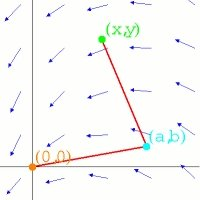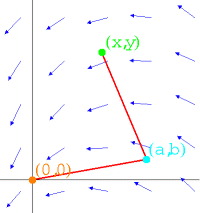# The planimeter

## Exhibit: table of content

##### Oliver Knill, SciCtr 434, knill@math.harvard.eduThe planimter connects is fixed at (0,0) has an "elbow" at (a,b) and points to (x,y). Both segments of length 1. Given (x,y), we can find (a,b) as a function of (x,y). The planimeter vector field F(x,y) = (-(y-b(x,y),x-a(x,y)) has curl 1. The weel rotation of the planimeter when (x(t),y(t)) traces a closed curve is the line integral of the vector field F along the curve. The key observation is that the field F has curl(F)=1 as can be verified with a simple computation (see the four lines of Mathematica below). By Greens theorem, the total wheel rotation is the area of the enclosed region R.
``` (* Mathematica verfies that the planimeter vector field has curl(F) = 1   *)